## Example Questions

### Example Question #1 : How To Find The Part From The Whole With Percentage

If n% of 400 is 260, then what is 20% of n?

25

14

31

13

15

13

Explanation:

n% of 400 is 260

A number as a percentage of a second number is equal to the first number divided by the second number, times 100%.

260 / 400 * 100% = .65 * 100% = 65%. n = 65.

20% of n?

20% of 65 is equal to 65 * 20 / 100 = 65 / 5 = 13.

### Example Question #2 : How To Find The Part From The Whole With Percentage

Auditions are being held for the annual school musical.  There are 18 roles available in the show.  If 75 students try out, what percentage of the students will get a role in the musical?

22%

28%

26%

30%

24%

24%

Explanation:

Percentage is #roles / # students = 18 / 75 = 0.24 = 24%

### Example Question #3 : How To Find The Part From The Whole With Percentage

You are making cookies and mixing the dry ingredients: flour, white sugar and brown sugar. If the recipe calls for 3 1/4 cups of flour, 1/2 cup of white sugar and 3/4 cup of brown sugar, what percentage of the recipe is white sugar?

17.5%

16.7%

20%

11.1%

10%

11.1%

Explanation:

Total ingredients = 3 1/4 cups + 1/2 cup + 3/4 cup = 4 1/2 cups

White sugar percentage = white sugar / total = (1/2)/(9/2) = .111 = 11.1%

### Example Question #4 : How To Find The Part From The Whole With Percentage

Jamie bought shoes for 30% off the regular price of \$37.99, rounded to the nearest cent. If she wanted to use exact change to pay, how much would she need in coins?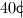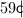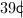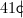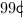Explanation: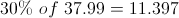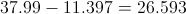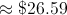### Example Question #5 : How To Find The Part From The Whole With Percentage

A class has 25 students. If 60% of them are boys, how many students are girls?

15

12

18

10

10

Explanation:

If 60% of the students are boys, 40% are girls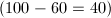. Multiply 25 by 40%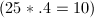. Therefore, 10 students are girls.

### Example Question #1 : How To Find The Part From The Whole With Percentage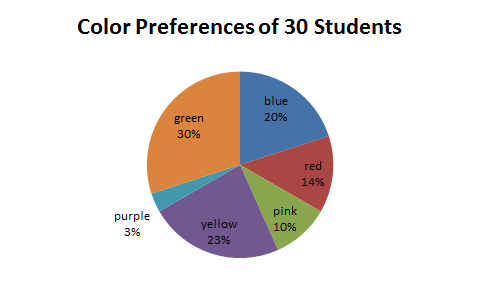The preceding graph depicts students' favorite colors. If there are 30 students in the class, how many prefer blue?

9

7

8

6

6

Explanation:

20% of the students prefer blue. We can calculate the number of students that prefer blue by multiplying the percentage by the total number of students.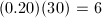### Example Question #7 : How To Find The Part From The Whole With Percentage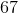is what part of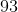?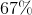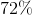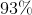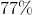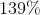Explanation:

To find how much percentis of, simply dividebyand multiply that answer by 100.

Thus,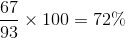### Example Question #8 : How To Find The Part From The Whole With Percentage

The Smith family consumed 35 pounds of table salt last year. As part of a healthier diet, they would like to cut their table salt consumption by 40% this year. In order to reach this goal, what is the maximum amount of salt, in pounds, that the Smith family can consume this year?

39

42

21

49

35

21

Explanation:

We need to first find 40 percent of the amount of table salt that the Smith family consumed in the previous year. In order to calculate this, we need to convert 40 percent into a decimal by moving the decimal place two spaces to the left and multiplying it by last year's salt consumption.Now, we need to subtract this amount from last year's salt consumption in order to calculate this years reduced consumption for the Smith family.